## F.1.1 Screened Interaction, Polarization, and Vertex Function

The equation (F.12) can be used as a starting point for a diagrammatic expansion. One possible way is to iterate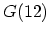in the functional derivative with respect to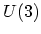, starting from the non-interacting GREEN's function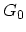. This procedure is described e.g. in , and specifically for the KELDYSH formalism, in . This expansion scheme is based on the non-interacting GREEN's function. In order to avoid the appearance of non-interacting GREEN's functions in the diagrammatic expansion without simultaneously complicating the rules for constructing the diagrams, one has to extend the equations for. Technically, this extension is based on the repeated change of variables and the consequent application of the chain-rule in the evaluation of the functional derivatives. One usually generates the following additional function
• the self-energy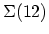, which contains information on both the renormalization of the single-particle energies and the scattering rates.
• the longitudinal polarization function, which describes the possible single-particle transitions as a result of a longitudinal electric field (which can either be an external field or the result of charge density fluctuations in the system),
• the screened COULOMB potential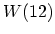, which differs from the bare COULOMB potential because of the possibility of single-particle transitions as described by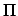, brought about by charge density fluctuations, and because of the related possibility of collective excitations,
• the vertex function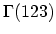, which serves to formally complete the set of equations.
Although the expanded set of functions still does not lead to a closed set of equations (an additional function,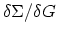, occurs), it allows for a perturbative solution by means of iterating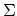in the derivative. The formal structure of these equations will turn out to be essentially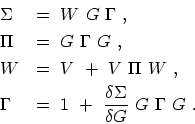(F.13)

By applying the chain rule for functional derivatives, one can introduce the derivative with respect to the effective potential. This allows one to write the self-energy (F.12) as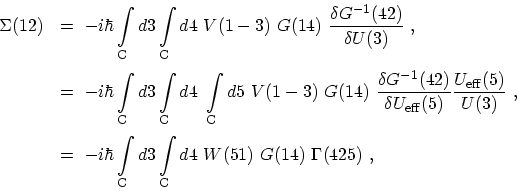(F.14)

where the screened interaction is defined as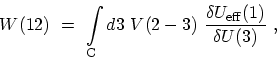(F.15)

and the vertex function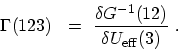(F.16)

Using the definition of the effective potential (F.7) together with (F.9) and the chain rule, the screened COULOMB potential, or equivalently, the inverse dielectric functionF.1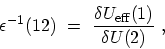(F.17)

can be written in terms of the polarization function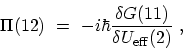(F.18)

in the following way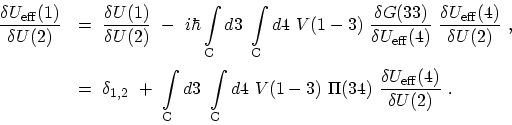(F.19)

In this way, one obtains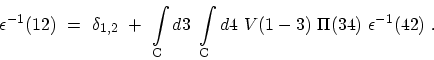(F.20)

and from (F.15)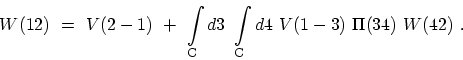(F.21)

By using the relation (F.10) one can express the polarization in terms of the vertex function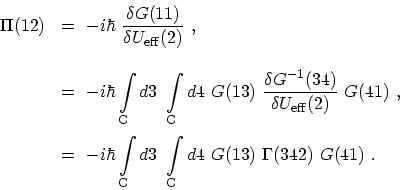(F.22)

The system of equations defining the self-energy is closed by the equation for the vertex functions. For that purpose one needs an explicit expression for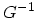in terms of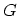. One can multiply and integrate both sides of the equation of motion (F.11) by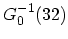and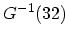, where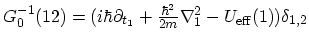. Finally, one obtains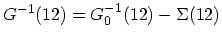, which can be used to rewrite the vertex function (F.16) as(F.23)

where the relation (F.10) has been used. Contributions proportional toare referred to as vertex corrections and describe interaction processes at the two-particle level. M. Pourfath: Numerical Study of Quantum Transport in Carbon Nanotube-Based Transistors